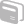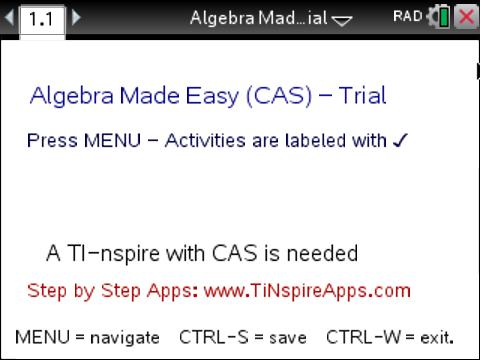# Activities

•• ##### Subject Area

• Math: Algebra I: Matrices
• Math: Algebra I: Quadratic Functions
• Math: Algebra I: Systems of Linear Equations
• Math: Algebra I: Equations
• Math: Precalculus: Systems of Equations
• Math: Geometry: Points, Lines and Angles

• ##### Author6-8
9-12
College

5-15 Minutes

• ##### Device
•TI-Nspire™ CX CAS/CX II CAS
• ##### Software

TI-Nspire™ CX CAS

4.2

• ##### Report an Issue

Algebra Made Easy - Trial Edition#### Activity Overview

In Algebra Made Easy - Trial Edition, students will use TI-Nspire technology to explore common algebra problems utilizing step-by-step processes.

#### Vocabulary

• Cramer's Rule
• Matrices
• Linear Equations
• Slope
• Midpoint
• Distance
• Points

#### About the Lesson

In "Algebra Made Easy- Trial Version", there are four different activities for students to explore.

To access the different activities within the TNS file, from the title screen, MENU >

• Algebra> (2) Solve 2x2 System

Solving Linear Equations Step by Step: Learn how to solve any 2x2 Linear System, Plug in the quadratic equation in the top box and view the steps to find its solution below.

• Quadratic Equations and Complex Numbers> (1) Do the Quadratic

Quadratic Equation Step by Step: Learn how to solve any quadratic equation, plug in the quadratic equation in the top box and view the steps below to find its solution below.

• Matrices> (8) Cramer Rule to Solve

Cramers Rule for Matrices Step-by-Step: Learn how to solve any linear system using Cramers Rule, plug in the matrices in the boxes and view the steps to find its solution below.

• Points and Lines> (1)Find Slope

Find Slope, Midpoint, Distance, given 2 points (x,y): Learn how to compute slope, midpoint, and distance step-by-step.

Note that all items with a check-mark next to it are functional in this trial version, so there are more activities available in the file to preview then those listed above. For more information on purchasing the full version, visit SMARTSOFT.